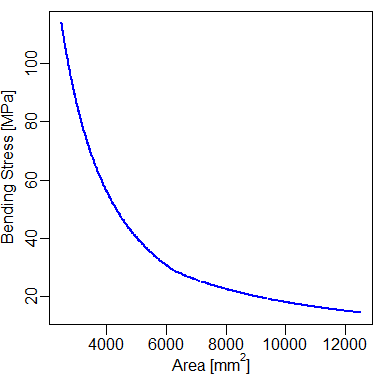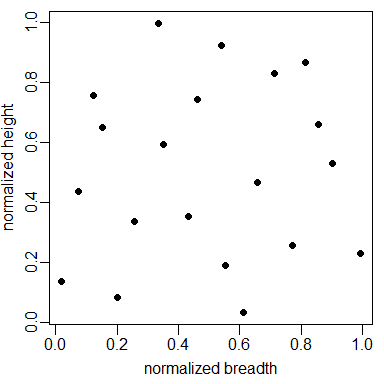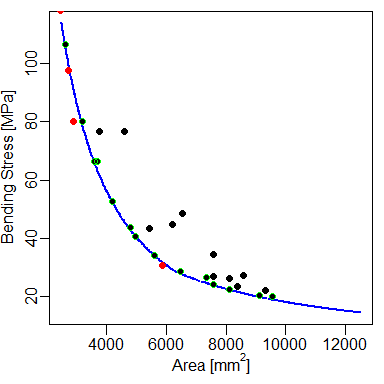Multiobjective Optimization of the Nowacki Beam

2020-06-01

In this paper, the well known multiobjective optimization problem: The Nowacki beam is solved. here, three different frameworks for dealing with many-objective problems using Kriging surrogate models are compared:

1. The efficient global optimization (EGO) algorithm applied to a single objective function of the combined objectives responses (MEGO);

2. The iterative maximization of the expected hypervolume improvement (EHVI);

3. A novel approach is also proposed here, the variance minimization of the Kriging-predicted Pareto front (VMKF).

To evaluate the efficiency of these three methods, a baseline solution is created by multiobjective direct optimization (no surrogates are used) applying the NSGA-II algorithm.

Introduction

Multiobjective optimization is a field of interest for many real-world applications. Usually, projects have multiple and conflicting goals and, most of the time, the relationship between the decision space (design variables) and the outcome is highly complex.

In the past years, Kriging have become one of the most popular surrogates on the industry (Forrester and Keane 2009). When using Kriging, usually the efficient global optimization (EGO) algorithm is the standard technique for single objective optimization. For costly multiple objectives, direct combination of the Kriging predictions and a multiobjective genetic algorithm (MOGA) can be used such as in (Li 2011). However, according to (Forrester and Keane 2009; Forrester, Sobester, and Keane 2008), there are currently two popular ways of constructing Pareto sets. The first approach, is to combine all goals into a single quantity and carry out the EGO algorithm. The weighting function have adjustable parameters that changes during the optimization problem so that the algorithm can potentially sweep all the objective space. For simplicity, here, this approach will be simply called MEGO. Another popular way to address many-objective problems is to generalize the expected improvement (EI) criterion into what is called the expected hypervolume improvement (EHVI) (Emmerich, Deutz, and Klinkenberg 2011; Shimoyama, Jeong, and Obayashi 2013). Although there are some efficient algorithms to calculate and/or estimate the expected hypervolume improvement such as (Hupkens, Emmerich, and Deutz 2014), it is usually a costly operation which significantly scales with the size of the Pareto set. To overcome this issue, a simpler, yet robust, algorithm is proposed by the present work. Here, each goal is modeled using Kriging then a state-of-the-art multiobjective algorithm (NSGA-II) is used to generate a Pareto set of the predicted mean of the surrogate models. From them, the design with higher value of predicted variance is chosen as an infill point.

Surrogate Multiobjetive Approaches

In the current work, three different Kriging-based multiobjective frameworks are studied, which are discussed in the following subsections. The derivation of the Kriging predictor and the design of experiments (DOE) concept are not covered in this paper. The reader can find a comprehensive mathematical description of these subjects in (Forrester, Sobester, and Keane 2008). The Kriging models where built using the R package DiceKriging (Roustant, Ginsbourger, and Deville 2012).

Multiobjective Efficient Global Optimization (MEGO)

EGO, proposed by Jones (Jones, Schonlau, and Welch 1998) for mono-objective optimization, consists in, from an initial set of samples $$\mathbf{X}$$, a Kriging model is built using the responses of a high-fidelity model, then the algorithm sequentially maximizes the expected improvement (EI) and updates the model at each iteration (including re-estimation of the hyperparameters).

The basic idea of the EI criterion is that by sampling a new point $$\mathbf{x^\star}$$ the results will be improved by $$y_\text{min} - y(\mathbf{x^\star})$$ if $$y(\mathbf{x^\star}) < y_\text{min}$$ or $$0$$ otherwise, where $$y_\text{min}$$ is the lowest value of $$\mathbf{y}$$ so far. Obviously, the value of this improvement is not known in advance because $$y(\mathbf{x^\star})$$ is unknown. However, the expectation of this improvement can be obtained using the information from the Kriging predictor. The EI criterion has important properties for sequential exploitation and exploration (filling) of the design space: it is null at points already visited (thus preventing searches in well-known regions and increasing the possibility of convergence); and at all other points it is positive and its magnitude increases with predicted variance (favoring searches in unexplored regions) and decreases with the predicted mean (favoring searches in regions with low predicted values).

The EGO algorithm can be easily imported to a multiobjective framework by creating a combined function of the qualities (Knowles 2006). The constrains of the optimization problem can be considered simply by building independent metamodels for each constraint and multiplying the EI of the composed objective function by the probability of each constraint to be met (Sasena, Papalambros, and Goovaerts 2002). The MEGO algorithm can be summarized as follows:

1. Generate an initial DOE $$\mathbf{X}$$ using an optimized Latin hypercube;
2. Evaluate $$\mathbf{X}$$ using high-fidelity models and store responses of $$\mathbf{f}=[f_1,f_2,\ldots,f_m]^T$$ and $$\mathbf{g}=[g_1,g_2,\ldots,g_p]^T$$ for the $$m$$-objectives and $$p$$-constraints. 1. while computational budget not exhausted do: a. Normalize the responses to fit in a hypercube of size $$[0,1]^m$$; a. For each $$\mathbf{x} \in \mathbf{X}$$ Compute a scalar quality by making $$f_{\mathbf{\lambda}} = \underset{i=1}{\overset{m}{\text{max}}}(\lambda_i f_i) + \rho \sum^m_{(i=1)}\lambda_i f_i$$, where $$\mathbf{\lambda}$$ is drawn uniformly at random from a set of evenly distributed unit vectors and $$\rho$$ is an arbitrary small value which we set to $$0.05$$; a. Build Kriging models for $$f_{\mathbf{\lambda}}$$ and for the constraints $$\mathbf{g}$$; a. Find $$\mathbf{x}^\star$$ that maximizes the constrained expected improvement: $$\mathbf{x}^\star = \text{arg}(\text{max}(\text{EI}_C(\mathbf{x})))$$;
1. Evaluate the true’’ values of $$f(\mathbf{x}^\star$$) and $$g(\mathbf{x}^\star)$$ using high-fidelity models and update the database.
3. end while.

Here, the EI is computed using a custom modified version of the functions provided on the R package DiceOptim (Ginsbourger et al. 2013) so that it could handle constrains. Also, on all approaches, the optimized Latin hypercube is built using the R package lhs (Carnell 2012).

Expected Hypervolume Improvement (EHVI)

For comparison, the expected hypervolume improvement (EHVI) is used as infill criterion. The EHVI is based on the theory of the hypervolume indicator (Zitzler and Thiele 1998), a metric of dominance of non-dominated solutions have. This metric consists in the size of the hypervolume fronted by the non-dominated set bounded by reference maximum points. I that sense, the EHVI is the expected improvement at the hypervolume size we would get by sampling a new point $$\mathbf{x^\star}$$. Here, the EHVI is computed using the R package GPareto (Binois and Picheny 2016). The EHVI function provided by this package do not account for constrains so a custom modification had to be implemented. The algorithm used here is similar to the MEGO and can be summarized as follows:

1. Generate an initial DOE $$\mathbf{X}$$ using an optimized Latin hypercube;
2. Evaluate $$\mathbf{X}$$ using high-fidelity models and store responses of $$\mathbf{f}=[f_1,f_2,\ldots,f_m]^T$$ and $$\mathbf{g}=[g_1,g_2,\ldots,g_p]^T$$ for the $$m$$-objectives and $$p$$-constraints.
3. while computational budget not exhausted do: a. Normalize the responses to fit a hypercube of size $$[0,1]^m$$; a. For each of the $$m$$-objectives and $$p$$-constraints, build a Kriging model; a. Find $$\mathbf{x}^\star$$ that maximizes the constrained expected hypervolume improvement: $$\mathbf{x}^\star = \text{arg}(\text{max}(\text{EHVI}_C(\mathbf{x})))$$; a. Evaluate the true’’ values of $$f(\mathbf{x}^\star$$) and $$g(\mathbf{x}^\star)$$ using high-fidelity models and update the database.
4. end while.

For this and the previous approach, the algorithm used to maximize the infill criteria ($$\text{EHVI}_C$$ and $$\text{EI}_C$$, respectively) is the one provided by the R package GenSA (???) which stands for generalized simulated annealing.

Variance Minimization of the Kriging-predicted Front (VMKF)

The proposed framework, VMKF, is based on the iterative improvement of the predicted Pareto set fidelity. Here, the idea is, from a given initial set of Kriging models (one for each cost or constraint function), to build a Pareto front using the predictor’s mean of each model as input functions. From the estimated front $$\mathbf{P}$$, the design with higher variance $$\mathbf{x}^\star$$ (i.e.: most isolated on the decision space) have it’s “true” value evaluated using the high fidelity models. A new set of Kriging models are then rebuilt and the process repeats until a stopping criteria is met. The proposed algorithm can be summarized as follows:

1. Generate an initial DOE $$\mathbf{X}$$ using an optimized Latin hypercube;
2. Evaluate $$\mathbf{X}$$ using high-fidelity models and store responses of $$\mathbf{f}=[f_1,f_2,\ldots,f_m]^T$$ and $$\mathbf{g}=[g_1,g_2,\ldots,g_p]^T$$ for the $$m$$-objectives and $$p$$-constraints.
3. while computational budget not exhausted do: a. For each of the $$m$$-objectives and $$p$$-constraints, build a Kriging model; a. Generate a Pareto set $$\mathbf{P}$$ using the mean predictor of the Kriging models using a state-of-art multiobjective optimization algorithm (such as NSGA-II); a. Find $$\mathbf{x}^\star \in \mathbf{P}$$ that maximizes the : $$\mathbf{x}^\star = \text{arg}(\text{max}(\text{s}_\text{km}(\mathbf{x})))$$; a. Evaluate the true’’ values of $$f(\mathbf{x}^\star$$) and $$g(\mathbf{x}^\star)$$ using high-fidelity models and update the database.
4. end while

Here, the NSGA-II implementation used is the one provided by the R package mco (Mersmann 2014).

The Nowacki Beam

However Kriging-based optimization is more useful for costly black box optimization problems, here we will demonstrate the technique using an analytic function for didactic proposes.

in the well known Nowacki beam optimization problem (Nowacki 1980), the aim is to design a tip loaded cantilever beam for minimum cross-sectional area and bending stress. The beam length is $$l=1500\;\text{mm}$$ and at is subject to a tip load force of $$F=5000\;\text{N}$$. The cross-section of the beam is rectangular, with breadth $$b$$ and height $$h$$, which are the design variables. The design is constrained by 5 requisites and the optimization problem can be formally defined as the following:

\begin{aligned} \text{find:} &\: \{b, h\}, \nonumber\\ \text{where:} &\: \{20 \leq h \leq 250\}, \nonumber\\ \text{and:} &\: \{10 \leq b \leq 50\}, \nonumber\\ \text{to minimize A:} &\: A = b\,h \nonumber\\ \text{and minimize B:} &\: \sigma = \frac{6Fl}{b^2h}, \nonumber\\ \text{subject to 1:} &\: \delta = \frac{12Fl^3}{Ebh^3} \leq 5, \\ \text{subject to 2:} &\: \sigma = \frac{6Fl}{b^2h} \leq 240, \nonumber\\ \text{subject to 3:} &\: \tau = \frac{3F}{2bh} \leq 120, \nonumber\\ \text{subject to 4:} &\: \text{AR} = \frac{h}{b} \leq 10, \nonumber\\ \text{subject to 5:} &\: F_\text{crit} = -\frac{4}{l^2}\sqrt{G\frac{(b^3h+hb^3)}{12}E\frac{b^3h}{12}\frac{1}{(1-v^2)}} \leq -2 F. \nonumber \end{aligned}

The material used on the original problem is a mild steel with a yield stress of $$\sigma_Y = 240 \text{MPa}$$, Young’s modulus $$E = 216.62 \text{GPa}$$, Poisson ratio $$\nu = 0.27$$ and shear modulus calculated as $$G = 86.65 \text{GPa}$$. For consistency, all values are physically interpreted on the unit system $$[\text{mm}$$, $$\text{N}$$, $$\text{MPa}]$$.

Quality Metric

Here, the quality of the Pareto sets found are compared using the inverted generational distance (IGD) metric (Shimoyama, Jeong, and Obayashi 2013). The IGD can be defined as $\text{IGD}(\mathbf{T},\mathbf{P}) = \frac{1}{|\mathbf{T}|} \sum_{\mathbf{t} \in \mathbf{T}} \text{min}(d(\mathbf{t} - \mathbf{p}))_{\mathbf{p} \in \mathbf{P}},$ where $$\mathbf{T}$$ and $$\mathbf{P}$$ are the true and the current Pareto sets, $$|\mathbf{T}|$$ is the number of designs in the true Pareto set and $$\mathbf{t}$$ and $$\mathbf{p}$$ are normalized vectors of length $$m$$ of the $$m$$-objectives of the true and the actual Pareto sets, respectively, and $$d(\quad)$$ is a distance metric that here is the Manhattan’s. Hence, IGD corresponds to the average distance between all designs in the true set and the closest design of the current set. Thus, the lower the IGD value, the better the method is. For the validation case, the “true” Pareto front (Fig. 1) is obtained by direct optimization using the NSGA-II algorithm using a population size of 500 and 100 generations, resulting in a near-uniform Pareto set of $$|\mathbf{T}| = 500$$.Methodology

First we load the moko package and the lhs package that we will use here for optimal DOE generation.

library(moko)
library(lhs)

After loading the necessary packages, we generate an initial DOE using an optimized Latin hypercube of n = 20 samples in two dimensions (d = 2) by doing:

n = 20
d = 2
set.seed(18)
doe <- optimumLHS(n,d)

The seed is arbitrary set to 100 so we can achieve reproducibility. This is how our sample looks:Now, we load the Nowacki beam function and compute the output.

fun <- nowacki_beam
res <- t(apply(doe, 1, fun))

The res object consists in a numeric matrix with 20 lines and 7 columns:

##           [,1]     [,2]    [,3]     [,4]     [,5]   [,6]         [,7]
##  [1,] 7583.035   34.584  -3.604 -205.416 -119.011 -6.117 -1241695.823
##  [2,]  708.964 1620.470 281.475 1380.470 -109.421 -7.836    -1671.848
##  [3,] 3615.173  171.235  11.312  -68.765 -117.925 -8.538  -323807.436
##  [4,] 2720.479   97.611  -1.011 -142.389 -117.243  0.556  -146710.229
##  [5,]  935.562 1770.643 446.351 1530.643 -111.983 -9.211   -19792.770
##  [6,] 1568.087  237.538   8.615   -2.462 -115.217 -0.692   -42131.351
##  [7,] 5445.722   43.243  -3.433 -196.757 -118.623 -3.295  -622053.908
##  [8,] 4609.511   76.800  -0.816 -163.200 -118.373 -6.495  -455763.448
##  [9,] 2763.636  161.186   6.049  -78.814 -117.286 -6.307  -156800.351
## [10,] 5860.066   30.740  -4.148 -209.260 -118.720  0.649  -717072.652
## [11,] 3758.513   76.615  -1.605 -163.385 -118.005 -3.503  -291288.284
## [12,] 9335.173   21.965  -4.307 -218.035 -119.197 -4.840 -1861194.422
## [13,] 1980.280  232.845  11.521   -7.155 -116.213 -5.190   -74432.481
## [14,] 7337.560   26.395  -4.213 -213.605 -118.978 -2.642 -1135366.662
## [15,] 3218.077  177.750  10.646  -62.250 -117.669 -8.077  -236050.920
## [16,] 2895.649   79.976  -2.150 -160.024 -117.410  3.040  -167268.564
## [17,]  554.691 1578.857 207.773 1338.857 -106.479 -5.240     3372.536
## [18,] 6527.316   48.697  -2.618 -191.303 -118.851 -6.929  -934550.979
## [19,] 2041.371  346.424  32.698  106.424 -116.326 -8.016   -88375.722
## [20,] 8114.381   26.309  -4.136 -213.691 -119.076 -4.524 -1400915.704

Each line of this matrix is a single design where the two first columns are the outputs that we need to maximize and the remaining columns are the constraints values. Any value that is grater than zero does not meet the constraint so the design is unfeasible. Note that, on this case, only the samples number 1, 4, 7, 8, 11, 12, 14, 18 and 20 are feasible. I does not matter right now, but we will check that latter, after fitting the model.

Now, we can create a multi-objective kriging model by calling the function mkm. Note that we need to setup the modelcontrol argument in order to tell the function that our data have two objectives. By doing that, the remaining columns of the response will be flagged as constraints. Also, in order to increase stability, we set the lower bounds for the kriging hyperparameter estimation as 0.1 for all variables (for more information check the Identifiability issues caused by large design interdistances section of (Roustant, Ginsbourger, and Deville 2012))

model <- mkm(doe, res, modelcontrol = list(objective = 1:2, lower = rep(0.1, d)))

The modelis an S4 objects with some usefull slots. For example, one can check which designs are feasible by simply calling:

which(model@feasible)
##   1  7  8 11 12 14 18 20

which returns the index of the feasible designs. Furthermore, one can get the feasible designs themselves by calling:

model@design[model@feasible,]
##          x.1       x.2
## 1  0.8548210 0.6590855
## 7  0.4624574 0.7438668
## 8  0.6565604 0.4657191
## 11 0.3512785 0.5924858
## 12 0.8134016 0.8672387
## 14 0.5394968 0.9232576
## 18 0.9026489 0.5285742
## 20 0.7123725 0.8295263

or the responses associated with those feasible designs with:

model@response[model@feasible,]
##         y.1      y.2        y.3       y.4       y.5       y.6        y.7
## 1  7583.035 34.58424 -3.6043393 -205.4158 -119.0110 -6.117252 -1241695.8
## 7  5445.722 43.24347 -3.4329719 -196.7565 -118.6228 -3.294710  -622053.9
## 8  4609.511 76.79969 -0.8163626 -163.2003 -118.3729 -6.494569  -455763.4
## 11 3758.513 76.61539 -1.6050900 -163.3846 -118.0045 -3.502522  -291288.3
## 12 9335.173 21.96469 -4.3069689 -218.0353 -119.1966 -4.840498 -1861194.4
## 14 7337.560 26.39487 -4.2133690 -213.6051 -118.9779 -2.642489 -1135366.7
## 18 6527.316 48.69679 -2.6181440 -191.3032 -118.8510 -6.929419  -934551.0
## 20 8114.381 26.30904 -4.1357378 -213.6910 -119.0757 -4.524183 -1400915.7

One can even filter only the feasible designs objective’s by:

model@response[model@feasible,model@objective]
##         y.1      y.2
## 1  7583.035 34.58424
## 7  5445.722 43.24347
## 8  4609.511 76.79969
## 11 3758.513 76.61539
## 12 9335.173 21.96469
## 14 7337.560 26.39487
## 18 6527.316 48.69679
## 20 8114.381 26.30904

This is only a small number of operations that can be done by using the slots of the mkm model. More details on the slots can be found on the help using ?'mkm-class'.

Now that we executed steps 1 and 2 for all optimization techniques presented here, we will apply the VMPF algorithm on the initial model to demonstrate how to handle the mkm object. Considering a total budget of 40 evaluations (which 20 were already spent building the initial model) we can code the technique as follows:

for (i in 21:40){
pred_ps <- predict_front(model, lower = rep(0,d), upper = rep(1,d))
pred_ps$sd <- predict(model, pred_ps$x)$norm_sd x_star <- pred_ps$x[which.max(pred_ps\$sd),]
y_star <- fun(x_star)
model <- mkm(
rbind(model@design, x_star),
rbind(model@response, y_star),
modelcontrol = model@control)
}

To check the IGD metric we first need to build a ps object from the actual data.

actual_ps <- ps(model@response[model@feasible,model@objective])
print(igd(actual_ps, true_ps))
##  0.0690039

Now we can visualize the actual Pareto front and check how good it is against the true front.Alternatively, one can use the VMPF function and save some coding lines. This function is basically a wrapper for the demonstrated algorithm, it receives a mkm model as input and returns the updated model after niter iterations. There are also wrappers for the other two algorithms that could be used as follows:

model <- mkm(doe, res, modelcontrol = list(objective = 1:2, lower = rep(0.1, d)))
niter <- 20
model.MEGO <- MEGO(model, fun, niter)
model.HEGO <- HEGO(model, fun, niter)
model.VMPF <- VMPF(model, fun, niter)